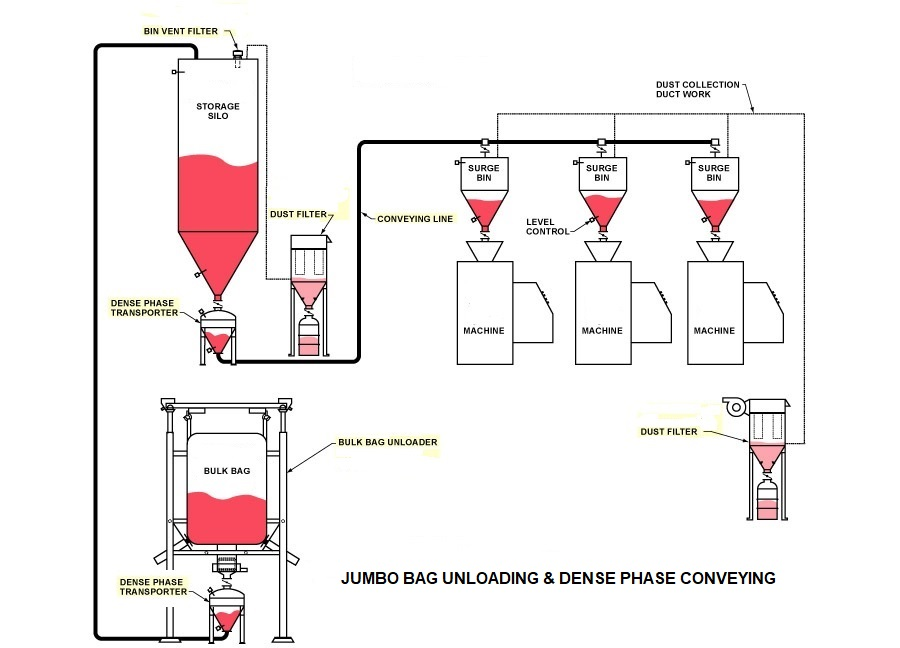Dense phase conveying system (calculation) is a method of transporting bulk materials such as powders, granules, and pellets using a low-velocity, high-pressure air stream. The goal of dense phase conveying is to move the material without damaging it and with minimal loss or degradation.The calculation of a dense phase conveying system typically involves several key factors, including:

1. -Material properties: The density, particle size, and flow characteristics of the material being conveyed are important factors in determining the design of the system.
2. Pipeline dimensions: The diameter and length of the pipeline are critical factors that must be carefully calculated to ensure that the system can operate effectively and efficiently.
3. Air flow rate: The amount of air required to transport the material through the pipeline must be calculated based on the material properties and pipeline dimensions.
4. Pressure drop: The pressure drop across the pipeline must be calculated to ensure that the system can maintain the required air flow rate.
5. Solids loading ratio: This is the ratio of the mass of material being transported to the mass of air used to transport it, and it is an important parameter in the design of a dense phase conveying system.

There are several software programs available that can assist in the calculation of dense phase conveying systems, such as PneuCalc and SysCAD. These programs use mathematical models and algorithms to predict the behavior of the material and air flow through the pipeline, allowing for accurate system design and optimization. It is important to note that the calculation of a dense phase conveying system requires a thorough understanding of the material being conveyed and the specific operating conditions of the system, and it should only be carried out by experienced professionals.

Here are the steps to calculate a dense phase conveying system:

1. Determine the bulk density of the material you want to convey. Bulk density is the weight of the material per unit volume. It is typically expressed in pounds per cubic foot (lb/ft3) or kilograms per cubic meter (kg/m3). You can find this information in the material safety data sheet (MSDS) or by performing a test.
2. Determine the desired conveying rate. This is the amount of material you want to move per unit of time. It is typically expressed in pounds per hour (lb/hr) or kilograms per hour (kg/hr). This information can be obtained from the process requirements.
3. Calculate the minimum conveying air velocity. The minimum conveying air velocity is the lowest air velocity required to move the material through the conveying line. It is typically expressed in feet per minute (ft/min) or meters per second (m/s). The minimum conveying air velocity is determined by the material’s bulk density and particle size. A commonly used equation for calculating the minimum conveying air velocity is:

Vmin = 29.8 x [(dp x ρp/ρg)^(1/2)],

where Vmin is the minimum conveying air velocity (ft/min or m/s), dp is the particle diameter (inches or millimeters), ρp is the material bulk density (lb/ft3 or kg/m3), and ρg is the density of the conveying gas (lb/ft3 or kg/m3).

1. Calculate the conveying air pressure. The conveying air pressure is the pressure required to generate the minimum conveying air velocity. It is typically expressed in pounds per square inch (psi) or kilopascals (kPa). A commonly used equation for calculating the conveying air pressure is:

P = 14.7 + (Vmin/CFM)^2 x (D/4)^2,

where P is the conveying air pressure (psi or kPa), Vmin is the minimum conveying air velocity (ft/min or m/s), CFM is the volumetric flow rate of air (cubic feet per minute or cubic meters per hour), and D is the diameter of the conveying line (inches or millimeters).

1. Calculate the conveying line diameter. The conveying line diameter is the diameter of the pipe or tube used to transport the material. It is typically expressed in inches or millimeters. The conveying line diameter is determined by the desired conveying rate and the conveying air velocity. A commonly used equation for calculating the conveying line diameter is:

D = [(4 x Q)/(π x Vmin)],

where D is the conveying line diameter (inches or millimeters), Q is the volumetric flow rate of material (cubic feet per minute or cubic meters per hour), and Vmin is the minimum conveying air velocity (ft/min or m/s).

1. Determine the length and layout of the conveying line. The length and layout of the conveying line are important factors in the performance of the system. The conveying line should be as short and straight as possible, with minimal bends or changes in direction. The line should also be sized appropriately to ensure that the material is conveyed at the desired rate.
2. Select the appropriate equipment. The equipment required for a dense phase conveying system includes a conveying vessel, a pressure vessel, a control valve, and a pressure regulator. The equipment should be selected based on the material properties, the desired conveying rate, and the system layout.
3. Test the system. Once

Translate »